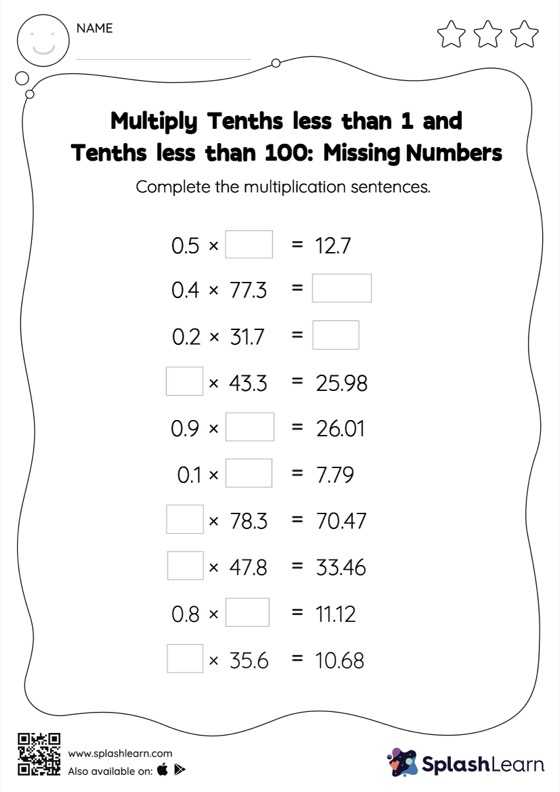# Multiply Tenths less than 1 and Tenths less than 100: Missing Numbers Worksheet

Home > Multiply Tenths less than 1 and Tenths less than 100: Missing NumbersCan your young mathematician multiply tenths less than 1 and tenths less than 100? That's exactly what they do here. A tenth multiplied by another tenth will result in a product with two decimal digits. Students use this idea in multiply tenths less than 1 and tenths less than 100 to find the missing number. In each problem, the numbers are laid out in the horizontal format. Students should try to use different strategies involving composing and decomposing numbers to solve these problems. This will help them develop flexibility and fluency.